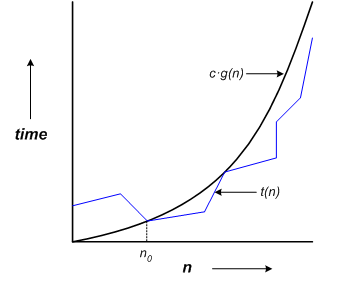# Timing Estimates

We can place an upper-bound on the execution time of algorithms using O(big-oh) notation. An algorithm that runs in O(n2) time indicates that execution time increases with the square of the dataset size. For example, if we increase dataset size by a factor of ten, execution time will increase by a factor of 100. A more precise explanation of big-oh follows.

Assume that execution time is some function t(n), wherenis the dataset size. The statement

t(n) = O(g(n))

implies that there exists positive constants c and n0such that

t(n) <= c·g(n)

for all n greater than or equal to n0. This is illustrated graphically in the following figure.Figure 1-4: Big-Oh

Big-oh notation does not describe the exact time that an algorithm takes, but only indicates an asymptotic upper bound on execution time within a constant factor. If an algorithm takes O(n2) time, then execution time grows no worse than the square of the size of the list.

Table 1-1: Growth Rates
n lgn n7/6 nlgn n2
1 0 1 0 1
16 4 25 64 256
256 8 645 2,048 65,536
4,096 12 16,384 49,152 16,777,216
65,536 16 416,128 1,048,565 4,294,967,296
1,048,576 20 10,568,983 20,971,520 1,099,511,627,776
16,777,216 24 268,435,456 402,653,183 281,474,976,710,656

Table 1-1 illustrates growth rates for various functions. A growth rate of O(lgn) occurs for algorithms similar to the binary search. The lg (logarithm, base 2) function increases by one whennis doubled. Recall that we can search twice as many items with one more comparison in the binary search. Thus the binary search is a O(lgn) algorithm.

If the values in Table 1-1 represented microseconds, then a O(n1.25) algorithm may take 10 microseconds to process 1,048,476 items, a O(lgn) algorithm 20 seconds, and a O(n2) algorithm up to 12 days! In the following chapters a timing estimate for each algorithm, using big-O notation, will be included. For a more formal derivation of these formulas you may wish to consult the references.